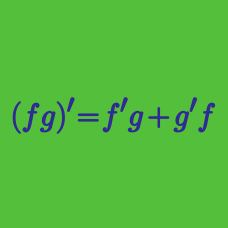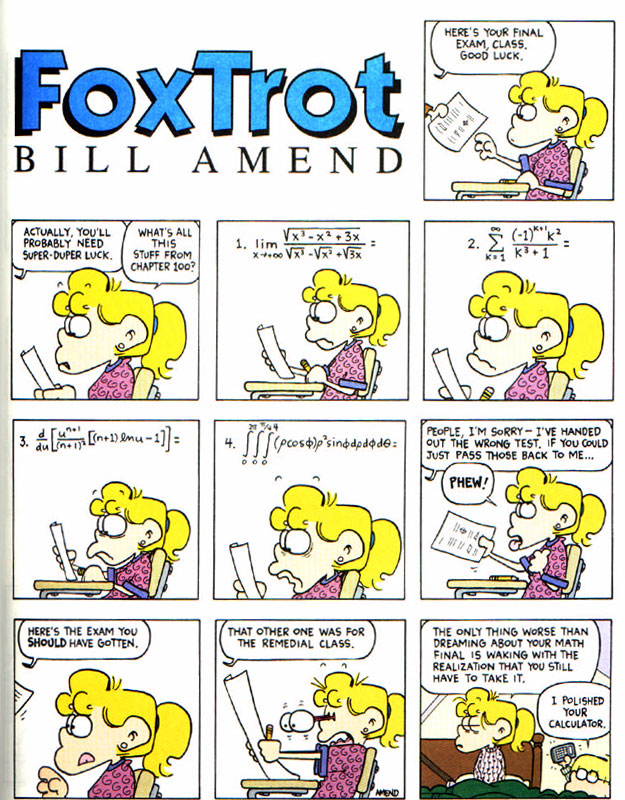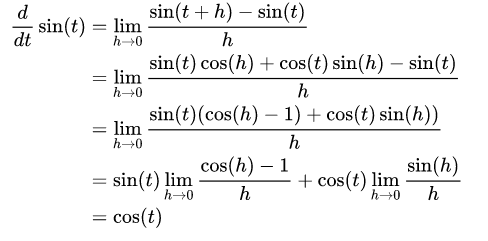Calculus

# Differentiation Rules: Level 2 ChallengesWhat is the following derivative equal to? $\dfrac {d}{du} \left[ \dfrac {u^{n+1}}{(n+1)^2} \cdot \left[ (n+1) \ln u - 1 \right] \right].$

What is the value of the derivative of $y = \left|\ln(x^2)\right|$ at $x = -\dfrac{1}{2}$?Above shows the derivative of $\sin(x)$ by the first principle.

What's the derivative of $\sin(x^{\circ})$?

If $\displaystyle\frac{d}{dx} f(x) = g(x)$ and $\displaystyle\frac{d}{dx}g(x) = f(x^2)$, then $\displaystyle\frac{d^2}{dx^2}f(x^3) =\ ?$

\begin{aligned} (A) &\quad f\left(x^6\right) & (B)&\quad g\left(x^3\right)\\ (C) &\quad 3x^2 g\left(x^3\right) & (D) &\quad 9x^4 f\left(x^6\right)+6x g\left(x^3\right)\\ (E) &\quad f\left(x^6\right) + g\left(x^3\right) & & \end{aligned}

Credit: 1969 AP Calculus AB Exam

$y = f(x), p = \dfrac{dy}{dx}, q = \dfrac{d^2y}{dx^2}$

What is $\dfrac{d^2x}{dy^2} \ ?$

×